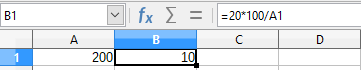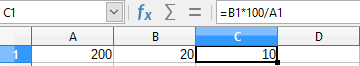In calc, how do i add number and percentage in the same cell ?

For example if in cell A1, I have entered 200 and in cell A2, I have entered 20, I want a display in cell A2 as 20(10%) to be calculated on its own.

That does not work like this. The cell contains either a value or a formula.

Either the value is included in the formula.Or the value is in a cell and the formula is separate.As @Hrbrgr has explained, you can’t do that in a spreadsheet. The concept of a spreadsheet is based on each cell containing a single “value”, each value being either a string of text, or a number, or a formula for calculating a number. What you want to do might be possible by devising a macro (a script that performs a series of steps) which would produce a string of text containing both the given number and the calculated number that you want. Complicated and no guarantees!